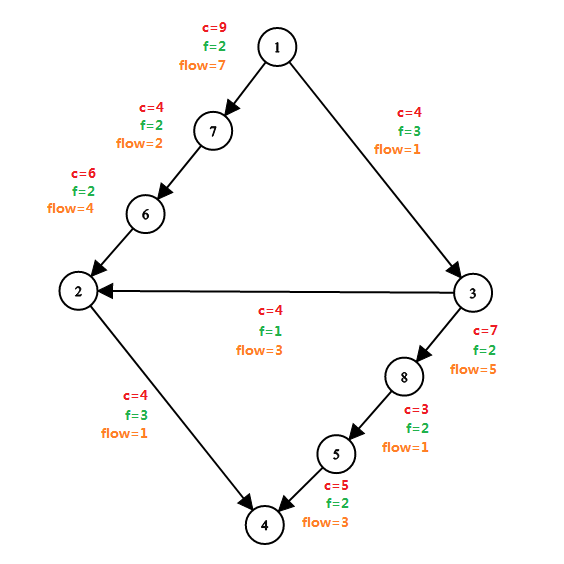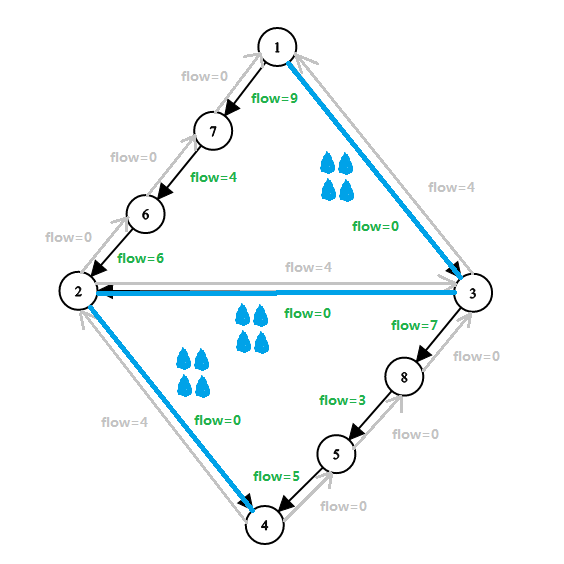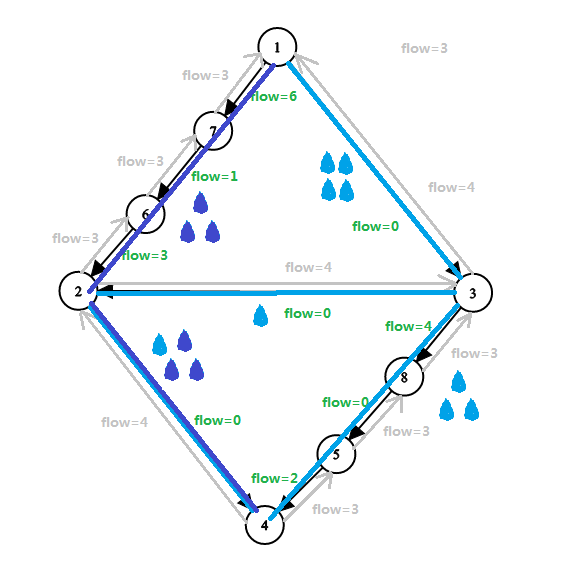# 【学习笔记】网络流算法简单入门

## 【大前言】

$QAQ$

## 一：【基本概念及性质】

### 【网络流基本概念】

• 网络流 $$(NetWork$$ $$Flow)$$ 一种类比水流的解决问题的方法。
（下述概念均会用水流进行解释）

• 网络 $$(NetWork)$$ 可以理解为拥有源点汇点有向图
（运输水流的水管路线路）

• $$(arc)$$ 可以理解为有向边。下文均用 “边” 表示。
（水管）

• 弧的流量 $$(Flow)$$ 简称流量。在一个流量网络中每条边都会有一个流量，表示为 $$f(x,y)$$ ，根据流函数 $$f$$ 的定义，$$f(x,y)$$ 可为负。
（运输的水流量）

• 弧的容量 $$(Capacity)$$ 简称容量。在一个容量网络中每条边都会有一个容量，表示为 $$c(x,y)$$
（水管规格。即可承受的最大水流量）

• 源点 $$(Sources)$$ 可以理解为起点。它会源源不断地放出流量，表示为 $$S$$
（可无限出水的 $$NB$$ 水厂）

• 汇点 $$(Sinks)$$ 可以理解为终点。它会无限地接受流量，表示为 $$T$$
（可无限收集水的 $$NB$$ 小区）

• 容量网络： 拥有源点汇点且每条边都给出了容量网络
（安排好了水厂，小区和水管规格的路线图）

• 流量网络： 拥有源点汇点且每条边都给出了流量网络
（分配好了各个水管水流量的路线图）

• 弧的残留容量： 简称残留容量。在一个残量网络中每条边都会有一个残留容量 。对于每条边，残留容量 $$=$$ 容量 $$-$$ 流量。初始的残量网络即为容量网络
（表示水管分配了水流量后还能继续承受的水流量）

• 残量网络： 拥有源点汇点且每条边都有残留容量网络残量网络 $$=$$ 容量网络 $$-$$ 流量网络
（表示了分配了一定的水流量后还能继续承受的水流量路线图）

（用 $$c$$ 表示容量，$$f$$ 表示流量，$$flow$$ 表示残留容量### 【网络流三大性质】

• 容量限制： $$\forall (x,y) \in E,f(x,y) \leqslant c(x,y)$$
（如果水流量超过了水管规格就爆了呀）

• 流量守恒： $$\forall x \in V且x \ne S且x \ne T,\sum_{(u,x) \in E}f(u,x) = \sum_{(x,v) \in E}f(x,v)$$
（对于所有的水管交界处，有多少水流量过来，就应有多少水流量出去，保证水管质量良好不会泄露并且不会无中生有）

• 斜对称性： $$\forall (x,y) \in E,f(y,x)=-f(x,y)$$
（可以暂且感性理解为矢量的正负。在网络流问题中，这是非常重要的一个性质）

$QAQ$

## 二：【最大流】

### 1.【概念补充】

• 网络的流量： 在某种方案下形成的流量网络汇点接收到的流量值。
（小区最终接收到的总水流量）

• 最大流： 网络的流量的最大值。
（小区最多可接受到的水流量）

• 最大流网络： 达到最大流流量网络
（使得小区接收到最多水流量的分配方案路线图）

### 2.【增广路算法 ( EK )】

#### 【概念补充】

• 增广路 $$(Augmenting$$ $$Path)$$ 一条在残量网络中从 $$S$$$$T$$ 的路径，路径上所有边的残留容量都为正。
（可以成功从水厂将水送到小区的一条路线）

• 增广路定理 $$(Augmenting$$ $$Path$$ $$Theorem)$$ 流量网络达到最大流当且仅当残量网络中没有增广路
（无法再找到一路线使得小区获得更多的流量了）

• 增广路方法 $$(Ford-Fulkerson)$$ 不断地在残量网络中找出一条从 $$S$$$$T$$增广路，然后根据木桶定律汇点发送流量并修改路径上的所有边的残留容量，直到无法找到增广路为止。该方法的基础为增广路定理，简称 $$FF$$ 方法。
（如果有一条路径可以将水运到小区里就执行，直到无法再运送时终止）

• 增广路算法 $$(Edmonds-Karp)$$ 基于增广路方法的一种算法，核心为 $$bfs$$ 找最短增广路，并按照 $$FF$$ 方法执行操作。增广路算法的出现使得最大流问题被成功解决，简称 $$EK$$ 算法。

#### 【算法流程】

$$(1).$$$$bfs$$ 找到任意一条经过边数最少的最短增广路，并记录路径上各边残留容量的最小值 $$cyf$$（残$$c$$$$y$$ $$flow$$）。 （木桶定律。众多水管一个也不能爆，如果让最小的刚好不会爆，其它的也就安全了）

$$(2).$$ 根据 $$cyf$$ 更新路径上边及其反向边的残留容量值。答案（最大流）加上 $$cyf$$

$$(3).$$ 重复 $$(1),(2)$$ 直至找不到增广路为止。

#### 【算法理解】

（不再显示容量和流量，用 $$flow$$ 表示残留容量，灰色边表示原图上的反向边，蓝色小水滴表示水流量）#### 【Code】

#include<algorithm>
#include<cstring>
#include<cstdio>
#include<queue>
#define Re register int
using namespace std;
const int N=1e4+3,M=1e5+3,inf=2e9;
struct QAQ{int to,next,flow;}a[M<<1];
inline void in(Re &x){
int f=0;x=0;char c=getchar();
while(c<'0'||c>'9')f|=c=='-',c=getchar();
while(c>='0'&&c<='9')x=(x<<1)+(x<<3)+(c^48),c=getchar();
x=f?-x:x;
}
inline int bfs(Re st,Re ed){
for(Re i=0;i<=n;++i)pan[i]=0;
h=1,t=0,pan[st]=1,Q[++t]=st,cyf[st]=inf;//注意起点cfy的初始化
while(h<=t){
Re x=Q[h++];
if(a[i].flow&&!pan[to=a[i].to]){//增广路上的每条边残留容量均为正
cyf[to]=min(cyf[x],a[i].flow);
//用cyf[x]表示找到的路径上从S到x途径边残留容量最小值
Q[++t]=to,pre[to]=i,pan[to]=1;//记录选择的边在链表中的下标
if(to==ed)return 1;//如果达到终点，说明最短增广路已找到，结束bfs
}
}
return 0;
}
inline void EK(Re st,Re ed){
while(bfs(st,ed)==1){
Re x=ed;maxflow+=cyf[ed];//cyf[ed]即为当前路径上边残留容量最小值
while(x!=st){//从终点开始一直更新到起点
Re i=pre[x];
a[i].flow-=cyf[ed];
a[i^1].flow+=cyf[ed];
x=a[i^1].to;//链表特性，反向边指向的地方就是当前位置的父亲
}
}
}
int main(){
in(n),in(m),in(st),in(ed);
EK(st,ed);
printf("%d",maxflow);
}


### 3.【Dinic】

$$EK$$ 算法中，每一次 $$bfs$$ 最坏可能会遍历整个残量网络，但都只会找出一条最短增广路

$$Dinic$$ 算法便提供了该思路的一种实现方法。

#### 【算法流程】

$$(1).$$ 根据 $$bfs$$ 的特性，找到 $$S$$ 到每个点的最短路径（经过最少的边的路径），根据路径长度对残量网络进行分层，给每个节点都给予一个层次，得到一张分层图

$$(2).$$ 根据层次反复 $$dfs$$ 遍历残量网络，一次 $$dfs$$ 找到一条增广路并更新，直至跑完能以当前层次到达 $$T$$ 的所有路径。

#### 【多路增广】

$$dfs$$ 时对于每一个点 $$x$$，记录一下 $$x \rightsquigarrow T$$ 的路径上往后走已经用掉的流量，如果已经达到可用的上限则不再遍历 $$x$$ 的其他边，返回在 $$x$$ 这里往后所用掉的流量，回溯更新 $$S \rightsquigarrow x$$ 上的信息。

#### 【当前弧优化】

（水管空间已经被榨干净了，无法再通过更多的水流，直接跳过对这些边的无用遍历）

#### 【时间复杂度分析】

（特别的，对于二分图$$Dinic$$ 最坏时间复杂度为 $$m\sqrt{n}$$

【Code】

#include<algorithm>
#include<cstring>
#include<cstdio>
#include<queue>
#define Re register int
using namespace std;
const int N=1e4+3,M=1e5+3,inf=2147483647;
struct QAQ{int to,next,flow;}a[M<<1];
inline void in(Re &x){
int f=0;x=0;char c=getchar();
while(c<'0'||c>'9')f|=c=='-',c=getchar();
while(c>='0'&&c<='9')x=(x<<1)+(x<<3)+(c^48),c=getchar();
x=f?-x:x;
}
inline int bfs(Re st,Re ed){//bfs求源点到所有点的最短路
h=1,t=0,dis[st]=1,Q[++t]=st;
while(h<=t){
Re x=Q[h++],to;
if(a[i].flow&&!dis[to=a[i].to]){
dis[to]=dis[x]+1,Q[++t]=to;
if(to==ed)return 1;
}
}
return 0;
}
inline int dfs(Re x,Re flow){//flow为剩下可用的流量
if(!flow||x==ed)return flow;//发现没有流了或者到达终点即可返回
Re tmp=0,to,f;
for(Re i=cur[x];i;i=a[i].next){
cur[x]=i;//当前弧优化cur=i
if(dis[to=a[i].to]==dis[x]+1&&(f=dfs(to,min(flow-tmp,a[i].flow)))){
//若边权为0，不满足增广路性质，或者跑下去无法到达汇点，dfs返回值f都为0，不必执行下面了
a[i].flow-=f,a[i^1].flow+=f;
tmp+=f;//记录终点已经从x这里获得了多少流
if(!(flow-tmp))break;
//1. 从st出来流到x的所有流被榨干。后面的边都不用管了，break掉。
//而此时边i很可能还没有被榨干，所以cur[x]即为i。
//2. 下面儿子的容量先被榨干。不会break，但边i成了废边。
//于是开始榨x的下一条边i'，同时cur[x]被更新成下一条边i'
//直至榨干从x上面送下来的水流结束（即情况1）。
}
}
return tmp;
}
inline void Dinic(Re st,Re ed){
Re flow=0;
while(bfs(st,ed))maxflow+=dfs(st,inf);
}
int main(){
in(n),in(m),in(st),in(ed);
Dinic(st,ed);
printf("%lld",maxflow);
}


### 4.【ISAP】

$$To$$ $$be$$ $$continued...$$

### 5.【HLPP】

$$To$$ $$be$$ $$continued...$$

### 4.【算法效率测试】

#### 【测试一】

$$(1).EK:$$$$(2).Dinic$$ $$+$$ 多路增广（喵？喵？喵？居然卡 $$Dinic$$！）$$:$$$$(3).Dinic$$ $$+$$ 多路增广 $$+$$ 当前弧优化 $$:$$#### 【测试二】

$$(1).EK:$$$$(2).Dinic$$ $$+$$ 多路增广 $$+$$ 当前弧优化 $$:$$$$(3).$$ 匈牙利算法$$QAQ$$ 好像混入了奇怪的东西）$$:$$$$To$$ $$be$$ $$continued...$$

### 5.【例题】

$QAQ$

## 三：【有上下界的最大流】

$$To$$ $$be$$ $$continued...$$

$QAQ$

## 四：【最小割】

### 1.【概念补充】

• 网络的割集$$(Network$$ $$Cut$$ $$Set)$$ 把一个源点$$S$$汇点$$T$$网络中的所有点划分成两个点集 $$s$$$$t$$$$S \in s,T \in t$$，由 $$x \in s$$ 连向 $$y \in t$$ 的边的集合称为割集。可简单理解为：对于一个源点$$S$$汇点$$T$$网络，若删除一个边集 $$E’ \subseteq E$$ 后可以使 $$S$$$$T$$ 不连通，则成 $$E’$$ 为该网络的一个割集
（有坏人不想让小区通水，用锯子割掉了一些边）

• 最小割 $$(Minimum$$ $$Cut)$$ 在一个网络中，使得边容量之和最小的割集
（水管越大越难割，坏人想要最节省力气的方案）

• 最大流最小割定理：$$(Maximum$$ $$Flow,Minimum$$ $$Cut$$ $$Theorem)$$ 任意一个网络中的最大流等于最小割【证明】
（可以感性理解为：最大流网络中一定是找出了所有的可行路径，将每条路径上都割掉一条边就能保证 $$S,T$$ 一定不连通，在此前提下每条路径上都割最小的边，其等价于最大流）

### 2.【最大权闭合子图】

$$To$$ $$be$$ $$continued...$$

### 3.【例题】

$QAQ$

## 六：【费用流】

### 1.【概念补充】

• 单位流量的费用 $$(Cost)$$ 简称单位费用。顾名思义，一条边的费用 $$=$$ 流量 $$\times$$ 单位费用。表示为 $$w(x,y)$$
（每根水管运一份水的花费。与$$“$$残留容量$$”$$的简化类似，通常直接称$$“$$费用$$”$$

• 最小费用最大流：最大流网络中，使得总费用最小。
（在运最多水的前提下，花钱最少）

### 2.【EK】

#### 【Code】

#include<algorithm>
#include<cstdio>
#include<queue>
#define LL long long
#define Re register int
using namespace std;
const int N=5003,M=5e4+3,inf=2e9;
struct QAQ{int w,to,next,flow;}a[M<<1];queue<int>Q;
inline void in(Re &x){
int f=0;x=0;char c=getchar();
while(c<'0'||c>'9')f|=c=='-',c=getchar();
while(c>='0'&&c<='9')x=(x<<1)+(x<<3)+(c^48),c=getchar();
x=f?-x:x;
}
inline int SPFA(Re st,Re ed){
for(Re i=0;i<=ed;++i)dis[i]=inf,pan[i]=0;
Q.push(st),pan[st]=1,dis[st]=0,cyf[st]=inf;
while(!Q.empty()){
Re x=Q.front();Q.pop();pan[x]=0;
if(a[i].flow&&dis[to=a[i].to]>dis[x]+a[i].w){
dis[to]=dis[x]+a[i].w,pre[to]=i;
cyf[to]=min(cyf[x],a[i].flow);
if(!pan[to])pan[to]=1,Q.push(to);
}
}
return dis[ed]!=inf;
}
inline void EK(Re st,Re ed){
while(SPFA(st,ed)){
Re x=ed;maxflow+=cyf[ed],mincost+=(LL)cyf[ed]*dis[ed];
while(x!=st){//和最大流一样的更新
Re i=pre[x];
a[i].flow-=cyf[ed];
a[i^1].flow+=cyf[ed];
x=a[i^1].to;
}
}
}
int main(){
in(n),in(m),in(st),in(ed);
EK(st,ed);
printf("%lld %lld",maxflow,mincost);
}


### 3.【Primal-Dual】

$$To$$ $$be$$ $$continued...$$

### 4.【ZKW 算法】

$$To$$ $$be$$ $$continued...$$

### 5.【算法效率测试】

$$To$$ $$be$$ $$continued...$$

### 6.【例题】

$QAQ$

## 七：【常见问题模型】

$$To$$ $$be$$ $$continued...$$

$QAQ$

## 八：【参考文献】

posted @ 2019-09-08 17:43  辰星凌  阅读(5427)  评论(7编辑  收藏  举报